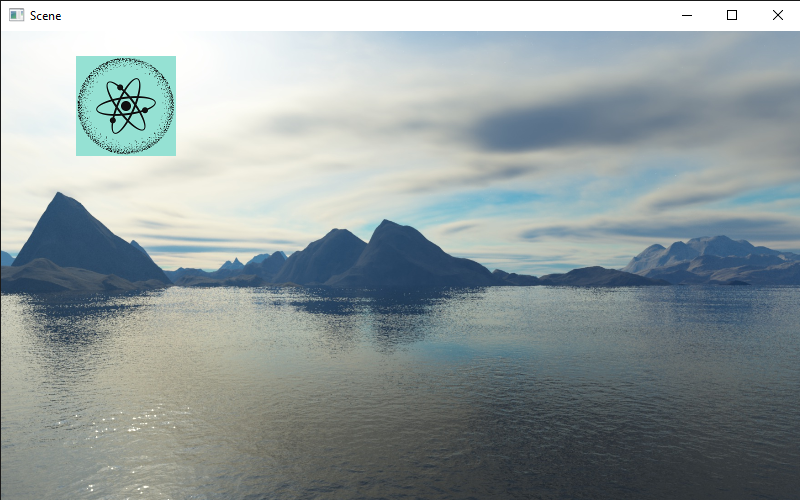Attention

You are viewing PyUnity docs under the develop branch. As such, they are only applicable if you installed from source. Go to https://docs.pyunity.x10.bz/en/latest/ for the most recent release.

# Tutorial 4: 2D¶

This tutorial we will be introducing many new components, namely the RectTransform and the Image2D. There is more to 2D than this, but most of the tutorial is quite dense in new ideas.

## Data types¶

To facilitate positioning objects, we are going to use RectAnchors and RectOffset. They both subclass RectData, which means they have two properties: min and max. They are both of type Vector2. For now, let’s ignore the RectAnchors.

### RectOffset¶

By ignoring RectAnchors we can simplify our offset to a literal rectangle. The min value specifies the top left corner of the rectangle, and the max value specifies the bottom right corner. In PyUnity, the X axis goes left to right and the Y axis goes top to bottom.

For example, a rect that is 100 pixels by 150 pixels, with a top left corner of (50, 75) would be like this:

>>> offset = RectOffset(
...     Vector2(50, 75),
...     Vector2(150, 225) # 100 + 50 and 150 + 75
... )


### RectTransform¶

A RectTransform has 5 notable properties: parent, anchors, offset, rotation and pivot. parent is a read-only property, which gets the RectTransform of its parent, if it has one. rotation is a float measured in degrees, and pivot is a point between (0.0, 0.0) and (1.0, 1.0) which defines the rotation point.

### Image2D¶

A RectTransform can’t really do much on its own, so we’ll look at the Image2D component. This renders a texture in the rect that is defined from the RectTransform. If you read tutorial 2, you may have used the Texture2D class. Here we can do the exact same:

>>> gameObject = GameObject("Image")
>>> transform.offset = RectOffset.Rectangle(
...     Vector2(100, 100), center=Vector2(125, 75))
>>> img.texture = Texture2D("python.png")


### Canvas¶

All 2D renderers must be a descendant of a Canvas element, which can customize the rendering of 2D components. We don’t need to worry about that too much, except that if we were to create an Image2D we must make it as a child or descendant of our canvas.

canvas = GameObject("Canvas")
img = GameObject("Image", canvas)
# And so on...


Here the second argument to the GameObject constructor specifies its parent, which must be a GameObject.

## Code¶

from pyunity import *

canvas = GameObject("Canvas")

gameObject = GameObject("Image", canvas)
transform.offset = RectOffset.Rectangle(
Vector2(100, 100), center=Vector2(125, 75))
img.texture = Texture2D("pyunity.png")



PyUnity image:This is the result:### Interaction¶

The easiest way to create an interactable image is to use the Button class. This will trigger whenever any part of the rect is clicked on. Here is an example:

class CallbackReceiver(Component):
def callback():
Logger.Log("Clicked")

# Same canvas and image code as above
...


Button.callback must be an Event object that contains a method of a component added to a GameObject. This is because when saving a PyUnity project, the Python code itself is not saved. It is also easier to reference a component and a method name in the saved scene file.

If you check the docs for the Button class, you can see two more attributes: state and button. This specifies what state and which button must be pressed for the callback to trigger.

If you would like more control over the button, using a Behaviour is easier as it can interact easily with other GameObjects and is created on a per-component basis. However, if you would like more interaction with the mouse, here is a method:

class HoverUpdater(Behaviour, GuiComponent):
def HoverUpdate(self):
Logger.Log("Hovering over component")

# Same canvas and image code as above
...


The GuiComponent class defines an abstract method called HoverUpdate which is called whenever the mouse is hovering over a component. This method will be called exactly once per canvas in a single GuiComponent each frame. In fact, this is how the Button class is implemented.

## Anchors¶

For a 2D rect to scale with the window, we can use the anchors property of the RectTransform. This has two values like the offset, a min and a max. These two values are between Vector2(0, 0) and Vector2(1, 1), where 0 and 1 represent the left and right of the window, or the top and bottom of the window. The offsets are applied where the anchors are.

The easiest way to understand this is when the anchors are a single point. For example, the default anchors are RectAnchors(Vector2(0, 0), Vector2(0, 0)). This means both points of the anchors are at Vector2(0, 0) so all offsets are calculated from the top left.

If we wanted our rect to be centered in the middle at all times, or be offset from the middle, we can set the anchors to be at Vector2(0.5, 0.5). Likewise, if we wanted our rect to be at the bottom right, we can use Vector2(1, 1).

This applies with two anchors: if we wanted our rect to be 50px away from each edge of the window, we would use anchors of RectAnchors(Vector2(0, 0), Vector2(1, 1)) and offset of RectOffset(Vector2(50, 50), Vector2(-50, -50)). This is how we can control the scaling of a rect with respect to the window size.

This tutorial was quite code-heavy, and it is not quite complete. If you are confused, please join our discord support server at https://discord.com/zTn48BEbF9.